# 1引言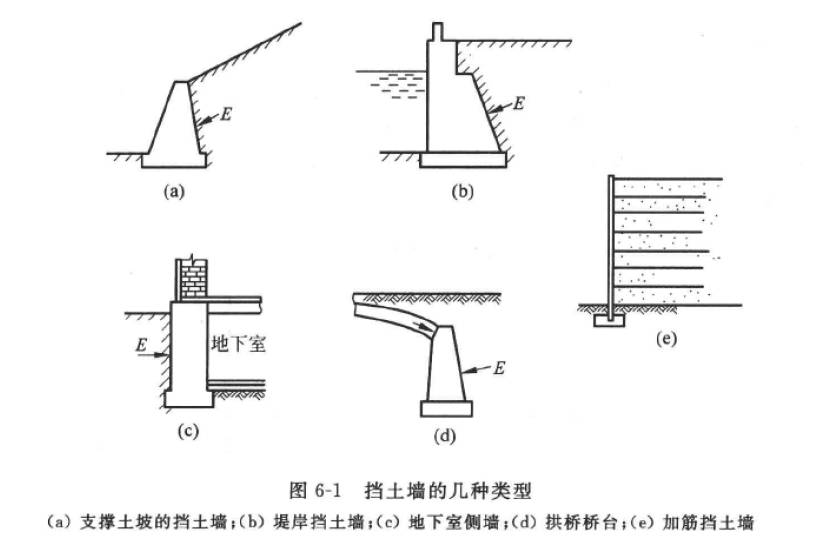1. 静止土压力，墙体受到侧向土压力之后，墙身变形或位移很小默认墙不发生变形，土体处于弹性平衡状态，此时的挡土墙受到的土压力为静止土压力，一般用$E_0$表示；

2. 主动土压力，挡土墙受墙后填土作用而发生背离填土方向移动或沿墙趾的转动，使得墙后填土达到极限平衡状态、此时，土体对挡土墙墙背的压力称为主动土压力，一般用$E_a$表示；

3. 被动土压力，挡土墙在巨大的外力作用下，向填土方向移动或转动，使墙后填土受到墙体挤压。当墙后土体达到极限平衡状态时，作用在挡土墙上的土压力称为被动土压力，用$E_p$表示。

# 2土压力的经典理论

## 2.1静止土压力理论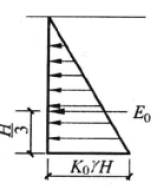$${\varepsilon _y} = \frac{{{\sigma _y}}}{E} - \frac{{\mu ({\sigma _x} - {\sigma _y})}}{E}$$

• 砂土：0.2~0.25
• 粘性土：0.25~0.4

$K_0$ 0.20 0.25 0.35 0.45 0.55

## 2.2朗肯土压力理论

1. 挡土墙墙背垂直、光滑；
2. 挡土墙墙后填土表面水平，土质均匀；
3. 墙背处各点均处于极限平衡状态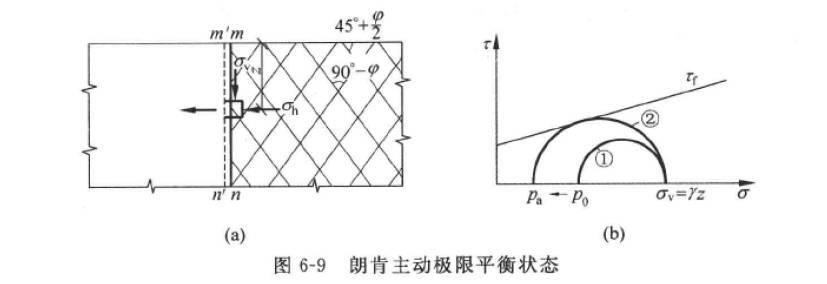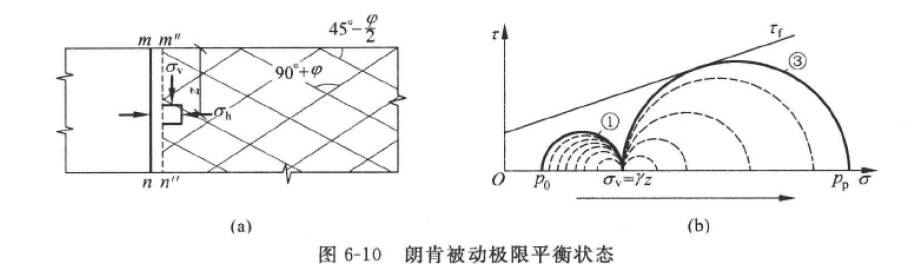$${P_a} = {K_a}\gamma z = \gamma z{\tan ^2}(45^\circ - \frac{\varphi }{2})$$

$${P_p} = {K_p}\gamma z = \gamma z{\tan ^2}(45^\circ + \frac{\varphi }{2})$$

$${P_a} = {K_a}\gamma z - 2c\sqrt {{K_a}} = \gamma z{\tan ^2}(45^\circ - \frac{\varphi }{2}) - 2c\tan (45^\circ - \frac{\varphi }{2})$$

$${P_p} = {K_p}\gamma z + 2c\sqrt {{K_p}} = \gamma z{\tan ^2}(45^\circ + \frac{\varphi }{2}) + 2c\tan (45^\circ + \frac{\varphi }{2})$$

$${P_a} = \gamma z\cos \beta \frac{{\cos \beta - \sqrt {co{s^2}\beta - {{\cos }^2}\varphi } }}{{\cos \beta - \sqrt {co{s^2}\beta - {{\cos }^2}\varphi } }}$$

$${P_a} = \gamma z\cos \beta \frac{{\cos \beta + \sqrt {co{s^2}\beta - {{\cos }^2}\varphi } }}{{\cos \beta - \sqrt {co{s^2}\beta - {{\cos }^2}\varphi } }}$$

## 2.3库伦土压力理论

1. 挡土墙是刚性的；
2. 挡土墙后填土为无粘性土；
3. 当挡土墙向前或向后偏移时，墙后滑动土楔体沿墙背和某个通过墙踵的平面发生滑动；
4. 滑动土楔体视为刚体，并处于极限平衡状态；$$\frac{E}{{\sin (\theta - \varphi )}} = \frac{W}{{\sin (180^\circ - (\theta - \varphi + \psi ))}}$$

$$W = \frac{{\gamma {H^2}}}{2} \times \frac{{\cos (\alpha - \beta )\cos (\theta - \alpha )}}{{{{\cos }^2}(\alpha )\sin (\theta - \alpha )}}$$

$$E = \frac{1}{2}\gamma {H^2}{K_a}$$

$${K_a} = \frac{{{{\cos }^2}(\varphi - \alpha )}}{{{{\cos }^2}\alpha \cos (\alpha + \delta )(1 + \sqrt {\frac{{\sin (\varphi + \delta )\sin (\alpha - \beta )}}{{\cos (\alpha + \delta )\cos (\alpha - \beta )}}} )}}$$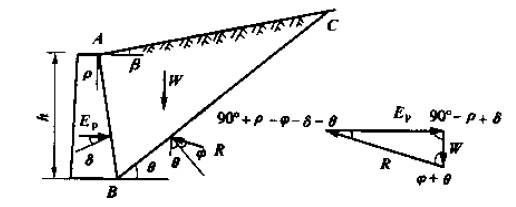$$E = W\frac{{\sin (\theta + \varphi )}}{{\sin (\theta + \varphi + \psi )}}$$

$${K_a} = \frac{{{{\cos }^2}(\varphi + \alpha )}}{{{{\cos }^2}\alpha \cos (\alpha - \delta )[1 + \sqrt {\frac{{\sin (\varphi + \delta )\sin (\alpha + \beta )}}{{\cos (\alpha - \beta )\cos (\alpha - \beta )}}} ]}}$$

1. 墙背土为无粘性土；
2. 滑裂面正好为经过墙踵的平面；
3. 墙后土层表面倾角小于土的内摩擦角；
4. 墙体与土接触一侧倾斜角较小时：
1. 当墙背向外倾斜过大时假定土楔体破裂面为平面会使计算得到的土压力减小到0；
2. 当墙背向内倾斜过大时滑动土体可能沿第二破裂面滑动。

# 3特殊情况下的土压力计算方法

## 3.1多层土层时的土压力计算方法

$$p_a = (\gamma_1 H1 + \gamma_2 * z) K_{a2}$$
$${E_{a2}} = ({\gamma _1}{H_{_1}}{H_2} + \frac{{{\gamma _2}H_2^2}}{2}){K_{a2}}$$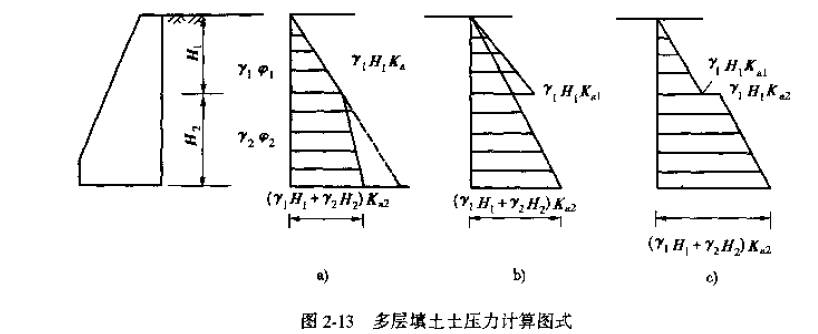## 3.2有限土体的土压力计算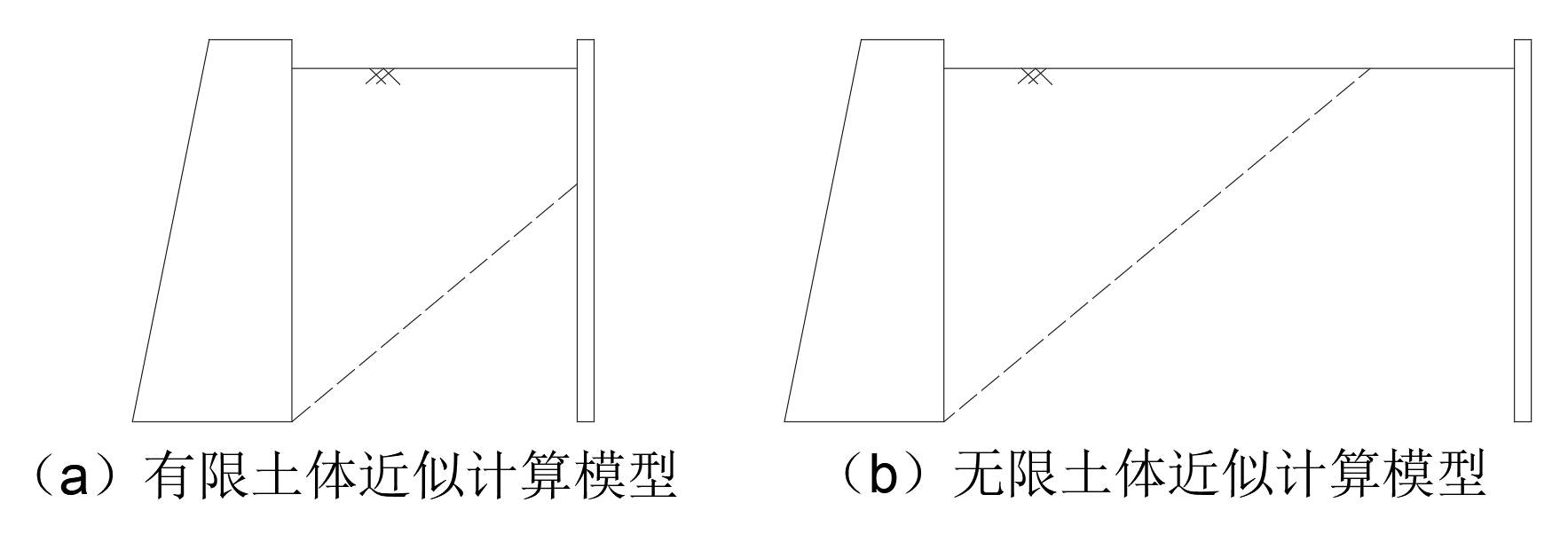### 3.2.1有限土体的主动土压力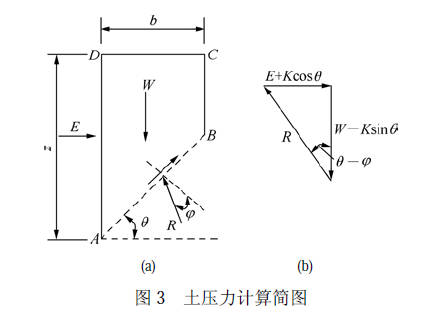$$W = \gamma b(z - \frac{b}{2}\tan \theta )$$

$$K = \frac{b}{{\cos \theta }}c$$

$$E = [\gamma b(z - \frac{b}{2}\tan \theta ) - bc\tan \theta ]\tan (\theta - \varphi ) - bc$$

$$\theta ' = \left\{ {\begin{array}{*{20}{c}} {\frac{1}{{2\gamma b\tan \varphi + 2c\tan \varphi }}[ - 2\gamma b}\\ { - 4c + 2({\gamma ^2}{b^2} + 4\gamma bc + 4{c^2} + 2{\gamma ^2}bz{{\tan }^3}\varphi }\\ { + 2{\gamma ^2}bz\tan \varphi + {\gamma ^2}{b^2}{{\tan }^2}\varphi + 4\gamma bc{{\tan }^2}\varphi }\\ { + 4\gamma zc{{\tan }^3}\varphi + 4\gamma zc\tan \varphi + 4{c^2}{{\tan }^2}\varphi {)^{\frac{1}{2}}}]} \end{array}} \right\}$$

### 3.2.2有限土体的被动土压力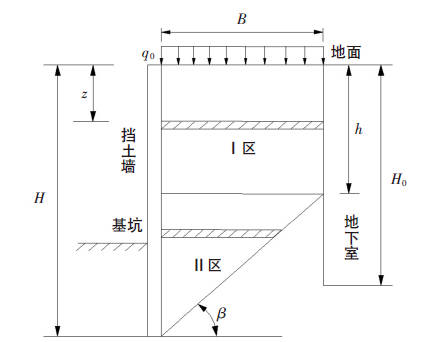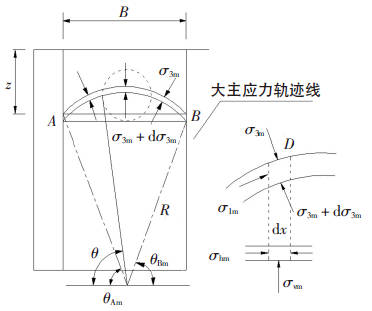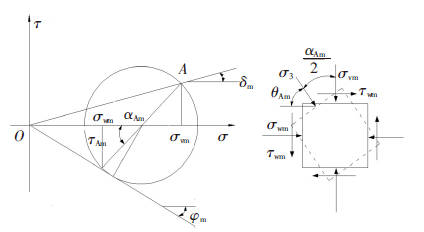$$\begin{array}{*{20}{l}} {{K_{pw1}}{\rm{ = }}\frac{{{\sigma _{wm}}}}{{{{\bar \sigma }_{vm}}}} = }\\ {\frac{{3(\cos {\theta _{Am}} + \cos {\theta _{Bm}})({{\cos }^2}{\theta _{Am}} + {K_{pm}}{{\sin }^2}{\theta _{Am}})}}{{3(\cos {\theta _{Am}} + \cos {\theta _{Bm}}) + ({K_{pm}} - 1)({{\cos }^3}{\theta _{Am}} + {{\cos }^3}{\theta _{Bm}})}}} \end{array}$$

$$\left\{ {\begin{array}{*{20}{c}} {{\theta _{Am}} = \frac{\pi }{2} - \frac{1}{2}[\arcsin (\frac{{\sin {\delta _m}}}{{\sin {\varphi _m}}}) + {\delta _m}]}\\ {{\theta _{Bm}} = \frac{\pi }{2} - \frac{1}{2}[\arcsin (\frac{{\sin {\alpha _m}}}{{\sin {\varphi _m}}}) + {\alpha _m}]} \end{array}} \right.$$

## 3.3地震作用下的土压力计算

1. 地震荷载简化为简单惯性力作用；
2. 墙后填土为刚体；
3. 土体内部处处均匀，各向同性。

$$P = C_z * k_H * G$$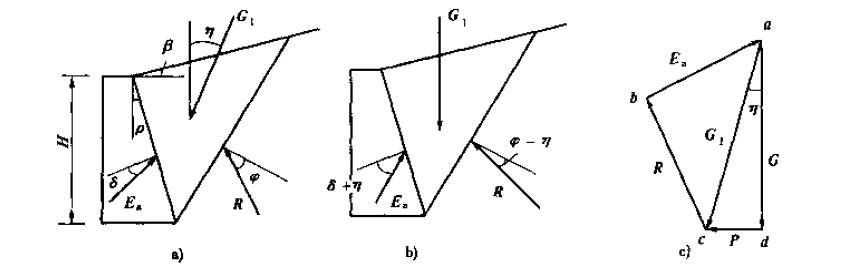$${E_a} = \frac{1}{2}\frac{\gamma }{{\cos \eta }}{H^2}{K_a}$$

## 3.4墙后土体有水时的土压力计算方法

$${p_{ak}} = {\sigma _{ak}}{K_{a,i}} - 2{c_i}\sqrt {{K_{a,i}}}$$
$${K_{a,i}} = {\tan ^2}(45 - \frac{{{\varphi _i}}}{2})$$
$${p_{pk}} = {\sigma _{pk}}{K_{p,i}} - 2{c_i}\sqrt {{K_{p,i}}}$$
$${K_{p,i}} = {\tan ^2}(45 + \frac{{{\varphi _i}}}{2})$$

$${p_{ak}} = ({\sigma _{ak}} - {u_a}){K_{a,i}} - 2{c_i}\sqrt {{K_{a,i}}} + {u_a}$$
$${p_{pk}} = ({\sigma _{pk}} - {u_p}){K_{p,i}} + 2{c_i}\sqrt {{K_{p,i}}} + {u_p}$$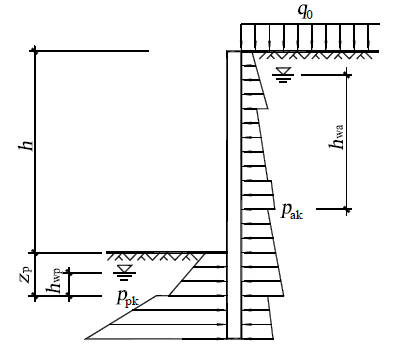# 4非极限状态下的土压力计算方法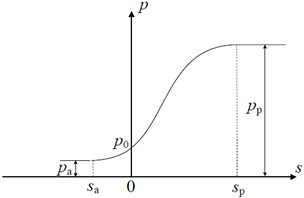## 4.1RB模式下的土压力计算方法

RB模式下的主动土压力计算公式：

$${p_h} = \left\{ {\begin{array}{*{20}{c}} {{K_1}\gamma z(0 \le z \le {h_1})}\\ {{K_2}\gamma (z - {h_1}) + {K_1}\gamma {h_1}({h_1} < z \le H)} \end{array}} \right.$$

$${K_1} = \frac{{\sin (\alpha - \varphi )\cos \delta \cot \alpha }}{{\cos (\alpha - \varphi - \varphi )}}$$
$${K_2} = \frac{{\cos (\alpha - \varphi + \delta )}}{{\cos (\alpha - \varphi - \delta )}}{K_1}$$

$${\alpha _{cr}} = \arctan \left[ {\sqrt {\frac{{\tan \varphi }}{{\tan (\varphi + \delta )}} + {{\tan }^2}(\varphi ) + \tan (\varphi )} } \right]$$

$${p_{RB}} = ({K_p} - {K_0})\gamma \left( {\frac{3}{{2 - 3m}}\frac{{{z^2}}}{H} - \frac{{3m}}{{2 - 3m}}z} \right) + {K_0}\gamma z$$

$${K_p} = \frac{{{{\cos }^2}\varphi }}{{{{(\sqrt {\cos \delta } - \sqrt {\sin (\delta + \varphi )\sin \varphi } )}^2}}}$$

## 4.2T变位模式下的土压力计算方法

T模式下的未达到极限状态和极限状态的被动土压力的计算公式：

$${p_T} = \left\{ {\begin{array}{*{20}{c}} {\left[ {\left( {{K_p} - K_0} \right)\frac{s}{{{s_p}}} + {K_0}} \right]\gamma z}&{s \le {s_p}}\\ {{K_p}\gamma z}&{s > {s_p}} \end{array}} \right.$$

RBT模式下的被动土压力计算公式如下式所示：

$${p_{RBT}} = \left\{ {\begin{array}{*{20}{c}} {{K_p}\gamma z}&{0 \le z \le (1 - \frac{{{s_p}}}{{{s_{\max }}}})H}\\ {\left( {{K_0} - ({K_0} - K{'_p})\frac{{{s_{\max }}}}{{{s_p}}}\frac{{H - z}}{H}} \right)\gamma z}&{(1 - \frac{{{s_p}}}{{{s_{\max }}}})H \le z \le H} \end{array}} \right.$$

$$K{'_p} = - \frac{2}{{1 + 3n}}{K_0} + \frac{{3 + 3n}}{{1 + 3n}}{K_p}$$

RTT模式下的被动土压力计算公式如下式所示

$${p_{RTT}} = \left\{ {\begin{array}{*{20}{c}} {(K{'_p} - {K_0})\frac{{z + nH}}{{(n + 1)H}}\frac{{{s_{\max }}}}{{{s_p}}}\gamma z + {K_0}\gamma z}&{{s_{\max }} \le {s_p}}\\ {\begin{array}{*{20}{c}} {\left( {{K_0} - ({K_0} - K{'_p})\frac{{{s_{\max }}}}{{{s_p}}}\frac{{z + nH}}{{(n + 1)H}}} \right)\gamma z}&{0 \le z \le (n + 1)\frac{{{s_p}}}{{{s_{\max }}}}H - nH}\\ {{K_p}\gamma z}&{(n + 1)\frac{{{s_p}}}{{{s_{\max }}}}H - nH \le z \le H} \end{array}}&{{s_{\max }} > {s_p}} \end{array}} \right.$$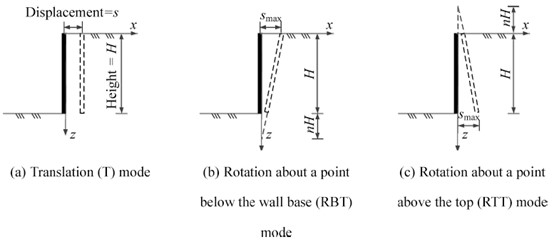## 4.3其他变位模式下的挡土结构线性变形土压力计算理论

1. 挡土墙刚性，只能绕某一点旋转，不会应为土压力变化产生扰度
2. 主动土压力和被动土压力与墙体位移的关系为线性关系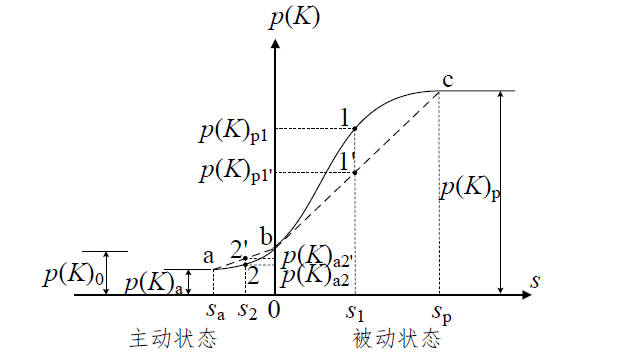# 5参考文献

1. JAKY I. The coefficient of earth pressure at rest [J]. Journal Soc of Hungarian Architects & Engineers, 1944
2. 公路桥涵设计通用规范[M]. 交通行业标准. 2015: 80p:A4.
3. 马平, 秦四清, 钱海涛. 有限土体主动土压力计算 [J]. 岩石力学与工程学报, 2008, S1): 3070-4.
4. 徐日庆, 徐叶斌. 考虑剪应力作用的有限土体被动土压力分析 [J]. 湖南大学学报(自然科学版), 2020, 47(09): 76-84.
5. 应宏伟, 张金红, 王小刚, et al. 有限土体刚性挡墙平动模式被动土压力试验研究 [J]. 岩土工程学报, 2016, 38(06): 978-86.
6. 中国建筑科学研究院, 中冶建筑研究总院有限公司, 华东建筑设计研究院有限公司, et al. 建筑基坑支护技术规程 [M]. 行业标准-建筑工业. 2012: 220P.;B5.
7. 汪刘军, 邓亚虹, 孙科, et al. 挡土墙地震土压力计算方法研究综述 [J]. 煤炭科学技术, 2018,
8. CONTE E, TRONCONE A, VENA M. A method for the design of embedded cantilever retaining walls under static and seismic loading [J]. Geotechnique, 2017, 67(12): 1081-9.
9. LIU, H. Required reinforcement stiffness for vertical geosynthetic-reinforced-soil walls at strength limit state [J]. Geotechnique, 2016,
10. MING-XI O U, LIU X R, SHI J X. Earth pressure applied in retaining wall of deep foundation pit considering influence of unloading and deformation [J]. Journal of Central South University(Science and Technology), 2012, 43(2): 669-74.
11. 范晓真. 非对称开挖内撑式支护受力变形机理及变形控制设计理论 [D]; 浙江大学, 2021.
12. FAN X-Z, XU C-J, LIANG L-J, et al. Analytical solution for displacement-dependent passive earth pressure on rigid walls with various wall movements in cohesionless soil [J]. Computers and Geotechnics, 2021, 140(104470.
13. 张恒志, 徐长节, 梁禄钜, et al. RB 模式下刚性挡墙有限土体主动土压力的 离散元模拟与理论研究 [J]. 岩土力学, 42(10): 2895-907.

https://www.eatrice.cn/post/ThreeKindsEarthPressure/

2022年4月20日